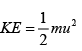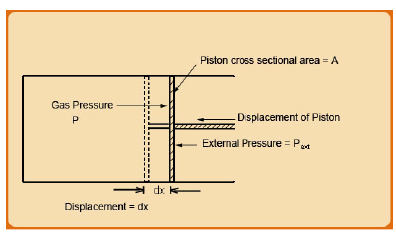Courses

# Types of Energies associated with Thermodynamic Processes Civil Engineering (CE) Notes | EduRev

## Civil Engineering (CE) : Types of Energies associated with Thermodynamic Processes Civil Engineering (CE) Notes | EduRev

The document Types of Energies associated with Thermodynamic Processes Civil Engineering (CE) Notes | EduRev is a part of the Civil Engineering (CE) Course Thermodynamics.
All you need of Civil Engineering (CE) at this link: Civil Engineering (CE)

Types of Energies associated with Thermodynamic Processes:

We know from the fundamentals of Mechanics, that the energy possessed by a body by virtue of its position or configuration is termed potential energy (PE). The potential energy of a body of mass m which is at an elevation z from the earth’s surface (or any particular datum) is given by: PE = mgz           ..(1.2)

Where, g is the acceleration due to gravity (= 9.81 m/s2).

The energy possessed by a body by virtue of its motion is called the kinetic energy (KE). For a body of mass m moving with a velocity u, the kinetic energy of the body is given by:.(1.3)

It follows that, like any mechanical body, a thermodynamic system containing a fluid, in principle may possess both PE and KE. It may be noted that both PE and KE are expressed in terms of macroscopic, directly measurable quantities; they, therefore, constitute macroscopic, mechanical forms of energy that a thermodynamic system may possess. As one may recall from the basic tenets of mechanics, PE and KE are inter-convertible in form.

It may also be noted that PE and KE are forms of energy possessed by a body as a whole by virtue of its macroscopic mass. However, matter is composed of atoms /molecules which have the capacity to translate, rotate and vibrate. Accordingly, one ascribes three forms intra-molecular energies: translational, rotational and vibrational.  Further, energy is also associated with the motion of the electrons, spin of the electrons, intra-atomic (nucleus-electron, nucleus-nucleus) interactions, etc. Lastly, molecules are also subject to inter-molecular interactions which are electromagnetic in nature, especially at short intermolecular separation distances. All these forms of energy are microscopic in form and they cannot be readily estimated in terms of macroscopically measurable properties of matter. It needs to be emphasized that the microscopic form of energy is distinct from PE and KE of a body or a system, and are generally independent of the position or velocity of the body. Thus the energy possessed by matter due to the microscopic modes of motion is referred to as the internal energy of the matter. The microscopic variety of energy forms the principal consideration in case of transformations that occur in a thermodynamic system. Indeed, as mentioned earlier, it is the realization that matter or fluids possessed useful form of microscopic energy (independent of macroscopic KE or PE) that formed the basis of the 18th century Industrial Revolution.

As we will see later, the majority of practical thermodynamic systems of interest are the ones that do not undergo change of state that entails significant change in its macroscopic potential and kinetic energies. Thus, it may be intuitively obvious that in a very general sense, when a thermodynamic system undergoes change of state, the attendant change in the internal energy is responsible for the energy leaving or entering the system. Such exchange of energy between a thermodynamic system and its surroundings may occur across the system boundary as either heat or work or both.

Thermodynamic Work:

Work can be of various forms: electrical, magnetic, gravitational, mechanical, etc. In general work refers to a form of energy transfer which results due to changes in the external macroscopic physical constraints on a thermodynamic system. For example, electrical work results when a charge moves against an externally applied electrical field. As we will see later, it is mechanical work that is most commonly encountered form in real thermodynamic systems, for example a typical chemical plant. In its simplest form, such work results from the energy applied to expand the volume of a system against an external pressure, or by driving a piston-head out of a cylinder against an external force. In both the last examples, work transfer takes place due to the application of a differential (or finite) force applied on the system boundary; the boundary either contracts or expands due to the application of such a force. In effect this results in the applied force acting over a distance, which results in mechanical energy transfer.

Consider the system in fig.1.2, where a force F acts on the piston and is given by pressure x piston area. Work W is performed whenever this force translates through a distance.Fig. 1.2 Illustration of Thermodynamic Work

Thus for a differential displacement ‘dx’ of the piston the quantity of work is given by the equation:

dW = Fdx                                               ..(1.4)

Here F is the force acting along the line of the displacement x. If the movement takes place over a finite distance, the resulting work is obtained by integrating the above equation. By convention, work is regarded as positive when the displacement is in the same direction as the applied force and negative when they are in opposite directions. Thus, for the above example, the equation 1.4 may be rewritten as:

dW = − PAd (Vt / A)

If the piston area ‘A’ is constant, then: dW = − PdVt         ..(1.6)

As may be evident from eqn. 1.6, when work is done on a system (say through compression) the volume decreases and hence the work term is positive. The reverse is true when the system performs work on the surroundings (through expansion of its boundary).

Heat

We invoke here the common observation that when a hot and a cold object are contacted, the hot one becomes cooler while the cold one becomes warmer. It is logical to argue that this need be due to transfer of ‘something’ between the two objects. The transferred entity is called heat. Thus, heat is that form of energy that is exchanged between system and its surrounding owing to a temperature differential between the two. More generally, heat is a form of energy that is transferred due to temperature gradient across space. Thus heat always flows down the gradient of temperature; i.e., from a higher to a lower temperature regions in space. In absence of such temperature differential there is no flow of heat energy between two points. Heat flow is regarded to be positive for a thermodynamic system, if it enters the latter and negative if it leaves.

Like work, heat is a form of energy that exists only in transit between a system and its surrounding. Neither work nor heat may be regarded as being possessed by a thermodynamic system. In a fundamental sense, the ultimate repositories of energy in matter are the atoms and molecules that comprise it. So after transit both work and heat can only transform into the kinetic and potential energy of the constituent atoms and molecules.

Offer running on EduRev: Apply code STAYHOME200 to get INR 200 off on our premium plan EduRev Infinity!

## Thermodynamics

33 videos|52 docs|36 tests

,

,

,

,

,

,

,

,

,

,

,

,

,

,

,

,

,

,

,

,

,

;